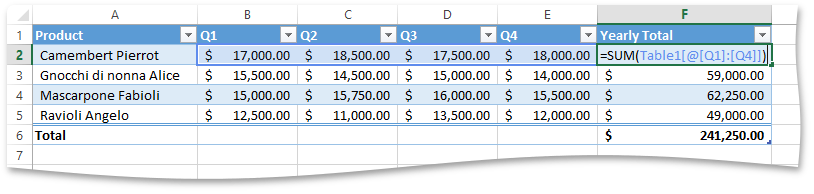.NET Framework 4.5.2+

# Use the Excel Export API to Create a Calculated Column

This example demonstrates how to create a calculated column. The latter uses a single formula that adjusts for each table row. To assign a formula to a table column, use the IXlTableColumn.SetFormula method. The formula you specify will be automatically filled into all cells of the table column.

Important

Due to the infrastructure of the Excel Export Library, which writes data directly into a stream in consecutive order, you should assign the required formula to a table column before you start generating any table data. Otherwise, a System.InvalidOperationException will be thrown.

The IXlTableColumn.SetFormula method allows you to specify a formula for a calculated column in different ways.

• Use textual representation of a formula

You can directly pass a string value representing a formula to be used to calculate column values to the IXlTableColumn.SetFormula method. A formula string should conform to the formula syntax rules and contain only supported elements (in particular, you cannot use structured references or defined names in your formula).

This is the most common and straightforward way to set a column formula. But to use it, you need to specify a formula parser to provide the capability to parse and validate string formulas. This can be done using the XlExport.CreateExporter method with the DevExpress.Spreadsheet.XlFormulaParser class instance passed as the parameter. Note that using the XlFormulaParser in your code requires a reference to the DevExpress.Spreadsheet.v22.2.Core.dll assembly.

• Compose a formula from tokens

Use the IXlTableColumn.SetFormula method with the XlExpression parameter to create a formula in a tokenized representation (a.k.a. a parsed expression). A parsed expression is a sequence of tokens arranged in Reverse-Polish notation, in which operands are followed by operators. Each token is represented by a descendant of the DevExpress.Export.Xl.XlPtgBase class. To create a formula of this kind, add the required tokens in the proper order to the XlExpression instance and pass it to the IXlTableColumn.SetFormula method as a parameter. However, this method of creating formulas is too complicated and you will rarely need to use it in your code.

• Construct a formula from the IXlFormulaParameter objects

This method enables you to construct a formula for a calculated column from a combination of the most commonly used functions (static methods of the XlFunc class), arithmetic and relational operators (static methods of the XlOper class) and constants (transformed into the IXlFormulaParameter object using the XlFunc.Param method). If your formula operates with table data, you can directly refer to specific ranges within the table by using structured references. A structured reference is represented by the XlTableReference class (which also implements the IXlFormulaParameter interface) and can be created by using the IXlTable.GetReference or IXlTable.GetRowReference method overloads.

This approach is demonstrated in the following example that creates a formula to calculate yearly sales for each product in the table and assigns it to the “Yearly Total” column.

``````IXlTable table;
// Specify an array containing column headings for a table.
string[] columnNames = new string[] { "Product", "Q1", "Q2", "Q3", "Q4", "Yearly Total" };

// Create the first row in the worksheet from which the table starts.
using (IXlRow row = sheet.CreateRow())
{
// Start generating the table with a header row displayed.
table = row.BeginTable(columnNames, true);
// Specify the total row label.
table.Columns.TotalRowLabel = "Total";
// Specify the function to calculate the total.
table.Columns.TotalRowFunction = XlTotalRowFunction.Sum;
// Specify the number format for numeric values in the table and the total cell of the "Yearly Total" column.
XlNumberFormat accounting = @"_([\$\$-409]* #,##0.00_);_([\$\$-409]* \(#,##0.00\);_([\$\$-409]* ""-""??_);_(@_)";
table.DataFormatting = accounting;
table.Columns.TotalRowFormatting = accounting;
// Set the formula to calculate annual sales of each product
// and display results in the "Yearly Total" column.
table.Columns.SetFormula(XlFunc.Sum(table.GetRowReference("Q1", "Q4")));
}

// Generate table rows and populate them with data.
using (IXlRow row = sheet.CreateRow())
row.BulkCells(new object[] { "Camembert Pierrot", 17000, 18500, 17500, 18000 }, null);
using (IXlRow row = sheet.CreateRow())
row.BulkCells(new object[] { "Gnocchi di nonna Alice", 15500, 14500, 15000, 14000 }, null);
using (IXlRow row = sheet.CreateRow())
row.BulkCells(new object[] { "Mascarpone Fabioli", 15000, 15750, 16000, 15500 }, null);
using (IXlRow row = sheet.CreateRow())
row.BulkCells(new object[] { "Ravioli Angelo", 12500, 11000, 13500, 12000 }, null);

// Create the total row and finish the table.
using (IXlRow row = sheet.CreateRow())
row.EndTable(table, true);
``````

The image below illustrates the result (the workbook is opened in Microsoft® Excel®).